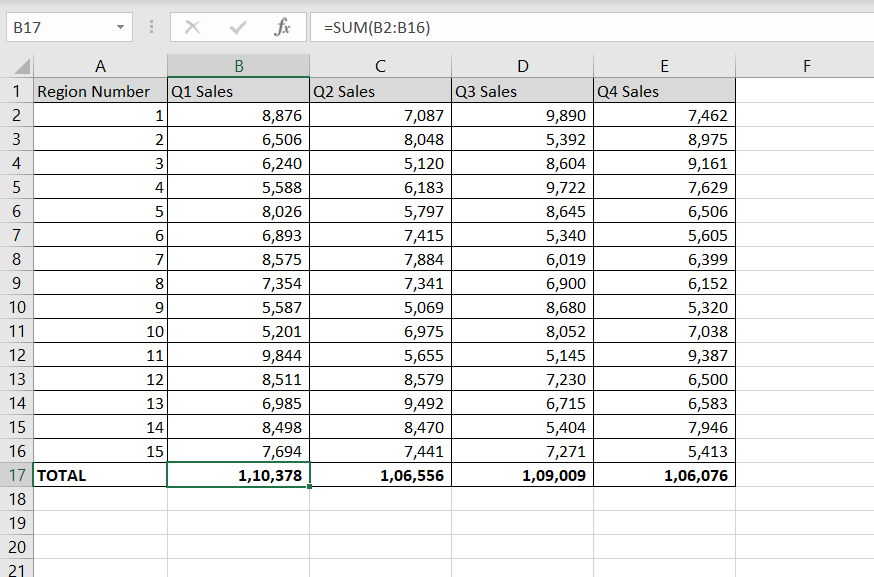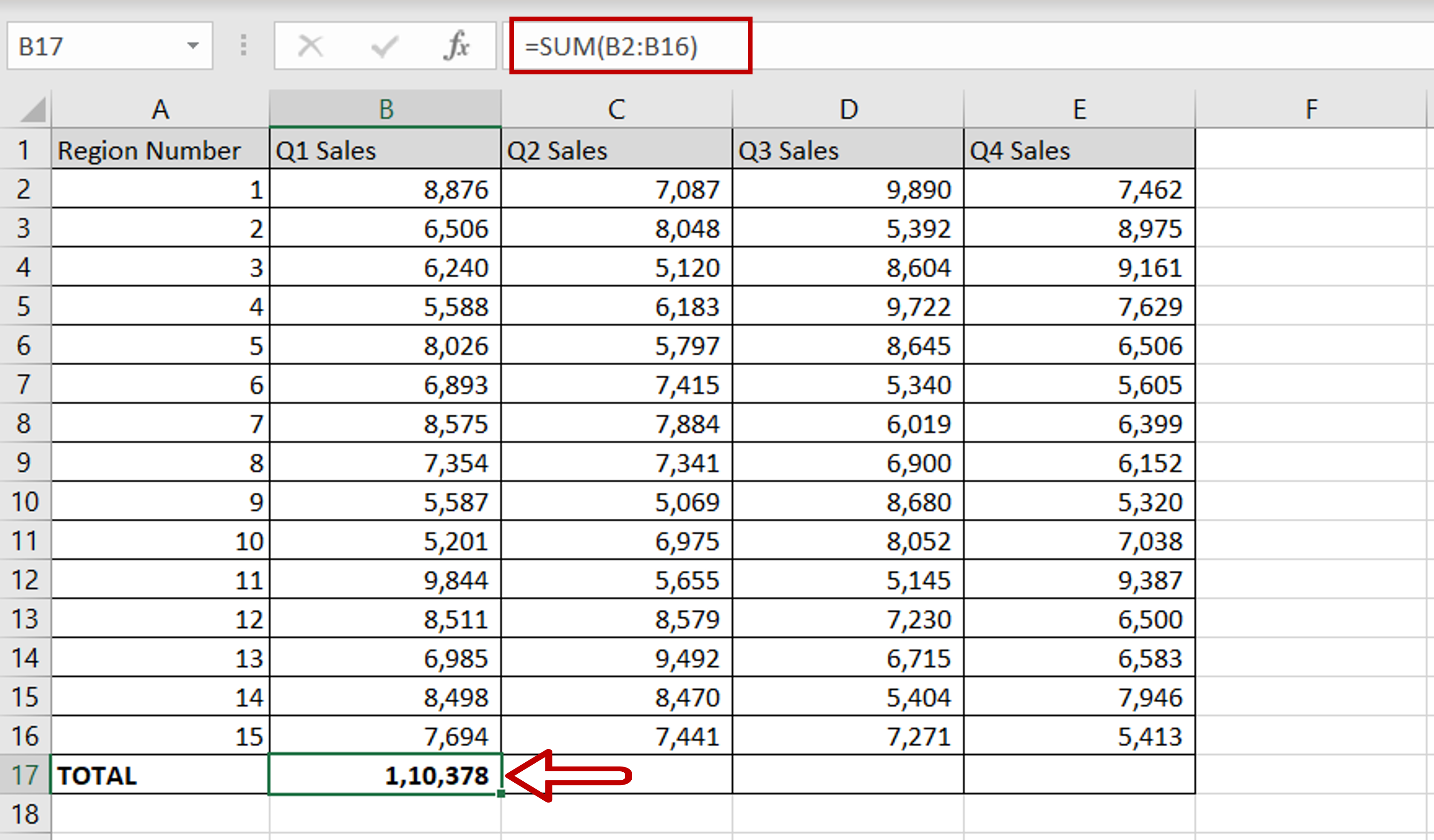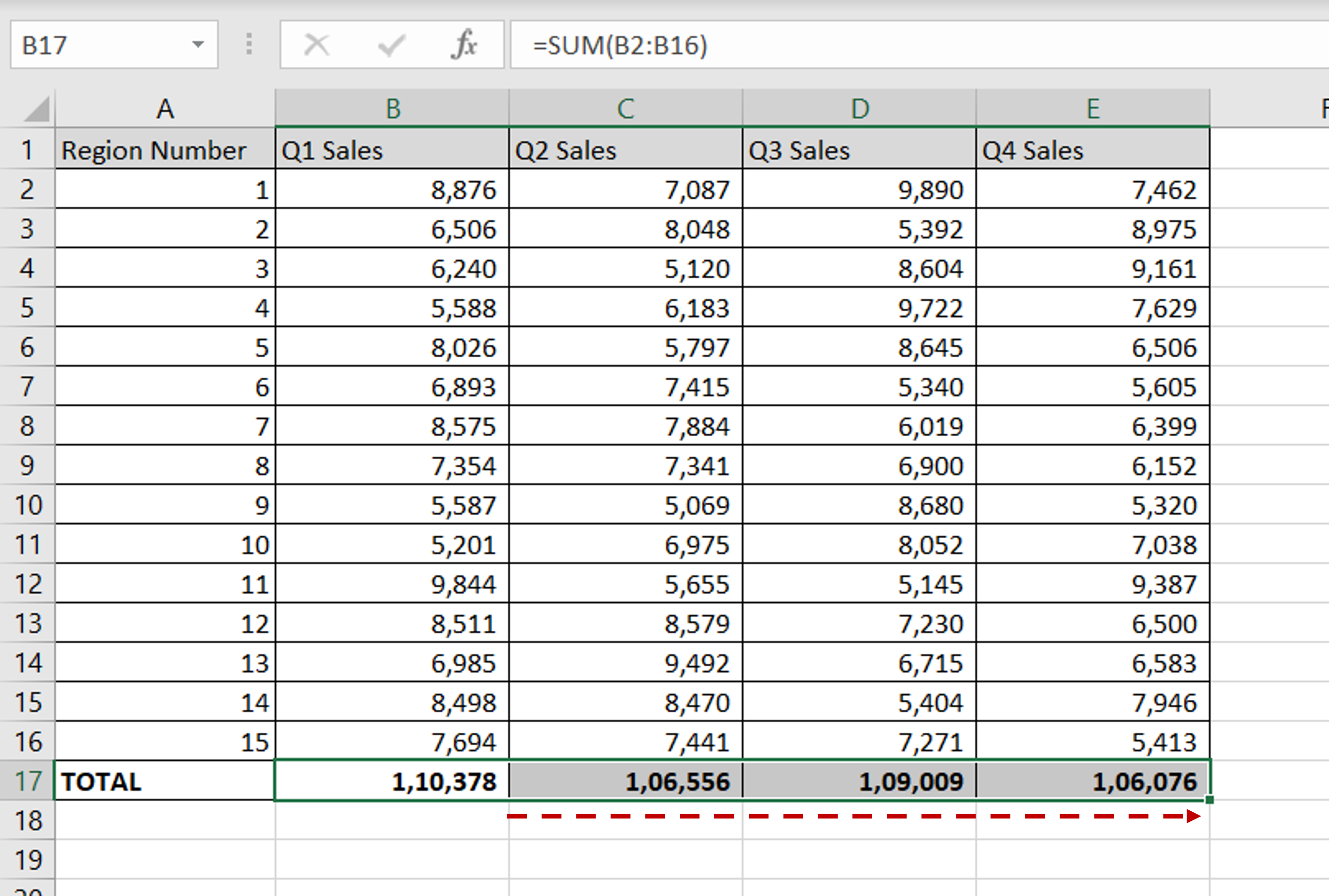# How do I add cells in Excel

You can watch a video tutorial here.Excel is widely used for calculations due to the several arithmetic operators and functions that it has. Instead of typing in numbers for calculations, you can use the cell references i.e. the address (Column header & Row number) of the cell in the calculation. When adding cells in Excel, you can do so using the cell reference.

### Step 1 – Use the SUM() function– In the destination cell, type the formula using the range of cells:
= SUM(Q1 Sales)

– Press Enter

### Step 2 – Copy the formula– Using the fill handle from the first cell, drag the formula to the remaining cells
OR
a) Select the cell with the formula and press Ctrl+C or choose Copy from the context menu (right-click)
b) Select the rest of the cells in the column and press Ctrl+V or choose Paste from the context menu (right-click